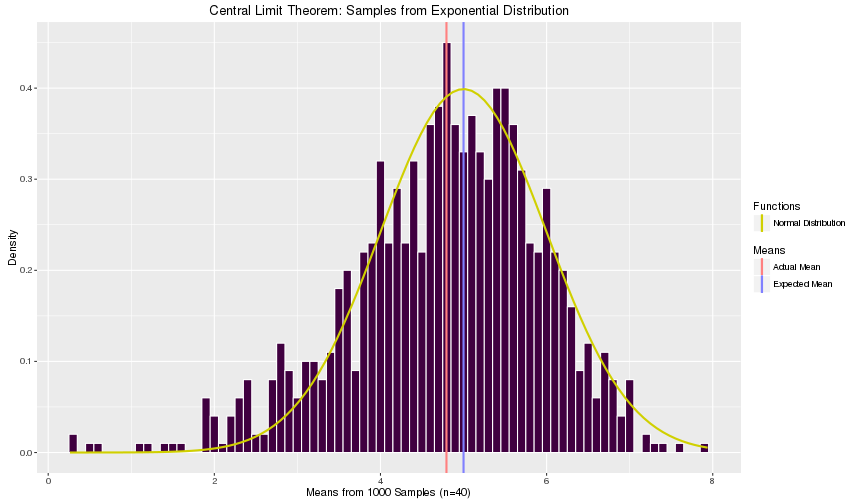# Central Limit Theorem on the Exponential Distribution

### Overview

The Central Limit Theorem states that when you take a series of samples from any distribution, the resulting distribution is normal. In this exercise we simulate taking 1000 samples of size 40 from the exponential distribution, calculate the theoretical means and variances, compare them to the simulated means and variances, and then plot the results.

### Simulations

To generate the simulations, we take a constant lambda of 0.2 for the exponential distribution and generate a series of means and adjust them according to the formula given by the Central Limit Theorem:

$\frac{\mathrm{Estimate - Mean\ of\ Estimate}}{\mathrm{Standard\ Error\ of\ Estimate}} = \frac{\bar{X}_{n}-\mu}{\sigma / \sqrt{n}}$

set.seed(43121)

means <- NULL
lambda <- 0.2
n <- 40
for(i in 1:1000){
vals <- rexp(n, rate=lambda)
means <- c(means, mean(vals))
}


### Sample Mean vs. Theoretical Mean

The expected mean of the exponential distribution is by definition $$\lambda^{-1}$$. Because we are generating a mean of a series of $$n$$ sample means, the expected mean of the sample distribution would be the sum of the expected values over $$n$$, which because the expected values of each mean is the expected mean times $$n$$, we get the formula:

$E(\bar{X}) = \frac{1}{n} \displaystyle\sum_{i=1}^{n} E(X_i) = \frac{1}{n} n \lambda^{-1} = \lambda^{-1}$

Comparing the actual mean to the expected mean, we can see that they are very close together.

actual_mean <- mean(means)
expected_mean <- 1/lambda
c(actual_mean, expected_mean)

##  4.979742 5.000000


### Sample Variance vs. Theoretical Variance

The expected standard deviation of the exponential distribution is by definition $$\lambda^{-1}$$. The expected variance of the sample means is the variance of the sum of the expected values of $$X$$ divided by $$n$$, which simplifies to:

$Var(\bar{X}) = Var(\displaystyle\sum_{i=1}^{n} \frac{E(X_i)}{n}) = \frac{1}{n^2}\displaystyle\sum_{i=1}^{n}Var(E(X_i)) = \frac{1}{n^2}n\sigma^2 = \frac{\sigma^2}{n} = \frac{1}{n\lambda^2}$

Same for the variance, comparing the actual variance to the expected variance, we can see that they are very close together.

actual_var <- var(means)
expected_var <- 1/(n*(lambda^2))
c(actual_var, expected_var)

##  0.6720464 0.6250000


### Distribution

Plotting the resulting adjusted sample means and comparing them to the standard normal curve, we can see that they follow the standard normal distribution.

library(ggplot2)
g <- ggplot(data=data.frame(means=adj_means), aes(x=means))
g <- g + ggtitle("Central Limit Theorem: Samples from Exponential Distribution")
g <- g + xlab("Means from 1000 Samples (n=40)") + ylab("Density")
g <- g + geom_histogram(
aes(y=..density..), fill="#400040", colour="#FFFFFF", binwidth=0.1
)
g <- g + geom_vline(
aes(xintercept=mean(means), colour="Actual Mean"), size=1,
show_guide=TRUE
)

## Warning: show_guide has been deprecated. Please use show.legend

g <- g + geom_vline(
aes(xintercept=1/lambda, colour="Expected Mean"), size=1,
show_guide=TRUE
)

## Warning: show_guide has been deprecated. Please use show.legend

g <- g + stat_function(fun=dnorm, args=list(mean=1/lambda),
aes(linetype="Normal Distribution"), colour="#D0D000", size=1,
show_guide=FALSE
)

## Warning: show_guide has been deprecated. Please use show.legend

g <- g + scale_colour_manual("Means", values=c(
"Expected Mean" = "#8080FF",
"Actual Mean" = "#FF8080",
"Normal Distribution" = NA
))
g <- g + scale_linetype_manual("Functions", values=c(
"Expected Mean" = "blank",
"Actual Mean" = "blank",
"Normal Distribution" = "solid"
))
g <- g + guides(
linetype = guide_legend(
override.aes = list(colour="#D0D000")
)
)
g